# Matrices - JEE Advanced Previous Year Questions with Solutions

JEE Advanced Previous Year Questions of Math with Solutions are available at eSaral. Practicing JEE Advanced Previous Year Papers Questions of mathematics will help the JEE aspirants in realizing the question pattern as well as help in analyzing weak & strong areas. eSaral helps the students in clearing and understanding each topic in a better way. eSaral also provides complete chapter-wise notes of Class 11th and 12th both for all subjects. Besides this, eSaral also offers NCERT Solutions, Previous year questions for JEE Main and Advance, Practice questions, Test Series for JEE Main, JEE Advanced and NEET, Important questions of Physics, Chemistry, Math, and Biology and many more. Download eSaral app for free study material and video tutorials.
Q. Let A be a 2 × 2 matrix Statement- $1: \operatorname{adj}(\operatorname{adj} A)=A$ Statement-2: $|$ adj $A|=| A |$ (1) Statement–1 is true, Statement–2 is false. (2) Statement–1 is false, Statement–2 is true. (3) Statement–1 is true, Statement–2 is true;Statement–2 is a correct explanation for Statement–1. (4) Statement–1 is true, Statement–2 is true; Statement–2 is not a correct explanation for statement–1. [AIEEE- 2009]
Ans. (4) Let $A=\left(\begin{array}{ll}{a} & {b} \\ {c} & {d}\end{array}\right)$ $\operatorname{adj}(\mathrm{A})=\left(\begin{array}{cc}{\mathrm{d}} & {-\mathrm{b}} \\ {-\mathrm{c}} & {\mathrm{a}}\end{array}\right)$ $\operatorname{adj}(\operatorname{adj} A)=\left(\begin{array}{ll}{a} & {b} \\ {c} & {d}\end{array}\right)=A$ statement is right Statement 2 We know $\mathrm{A}$ adj $(\mathrm{A})=|\mathrm{A}| \mathrm{I}_{\mathrm{n}}$ taking determinant $|\mathrm{A} \cdot \operatorname{adj}(\mathrm{A})|=\| \mathrm{A}\left|\mathrm{I}_{\mathrm{n}}\right|$ $\Rightarrow|\operatorname{adj}(\mathrm{A})|=|\mathrm{A}|^{\mathrm{n}-1}$ Here $\mathrm{n}=2$ (order) so $|\operatorname{adj} \mathrm{A}||\mathrm{A}|^{2-1}=|\mathrm{A}|$ so statement 2 is also true and 2 is not explanation for statement 1
Q. The number of 3× 3 non-singular matrices, with four entries as 1 and all other entries as 0, is :- (1) Less than 4            (2) 5             (3) 6              (4) At least 7 [AIEEE-2010]
Ans. (4)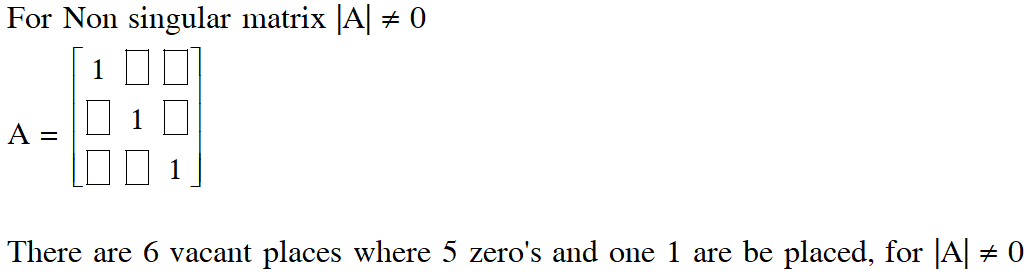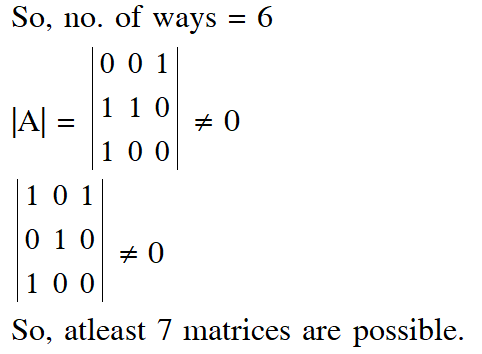Q. Let A be a $2 \times 2$ matrix with non-zero entries and let $\mathrm{A}^{2}=\mathrm{I},$ where I is $2 \times 2$ identity matrix. Define $\operatorname{Tr}(\mathrm{A})=$ sum of diagonal elements of $\mathrm{A}$ and $|\mathrm{A}|=$ determinant of matrix $\mathrm{A}$. Statement- $1: \operatorname{Tr}(\mathrm{A})=0$ Statement-2: $|\mathrm{A}|=1$ (1) Statement–1 is true, Statement–2 is true; Statement–2 is a correct explanation for Statement–1. (2) Statement–1 is true, Statement–2 is true; Statement–2 is not a correct explanation for statement–1. (3) Statement–1 is true, Statement–2 is false. (4) Statement–1 is false, Statement–2 is true. [AIEEE-2010]
Ans. (3) Statement 1: Let $\mathrm{A}=\left(\begin{array}{ll}{\mathrm{a}} & {\mathrm{b}} \\ {\mathrm{c}} & {\mathrm{d}}\end{array}\right) \mathrm{a}, \mathrm{b}, \mathrm{c}, \mathrm{d},$ are non zero $A^{2}=\left(\begin{array}{ll}{a} & {b} \\ {c} & {d}\end{array}\right)\left(\begin{array}{ll}{a} & {b} \\ {c} & {d}\end{array}\right)=\left(\begin{array}{ll}{1} & {0} \\ {0} & {1}\end{array}\right)$ $\Rightarrow a^{2}+b c=1$ $\Rightarrow \mathrm{ab}+\mathrm{bd}=0 \Rightarrow \mathrm{b}(\mathrm{a}+\mathrm{d})=0$ so $\mathrm{b} \neq 0,(\mathrm{a}+\mathrm{d})=0$ $a+d=0 \Rightarrow \operatorname{tr}(A)=0$ Statement 2: $|A|=a d-b c=-a^{2}-b c=-\left(a^{2}+b c\right)=-1$ So Statement 2 is false.
Q. Let A and B be two symmetric matrices of order 3. Statement-1 : A(BA) and (AB)A are symmetric matrices. Statement-2 : AB is symmetric matrix if matrix multiplication of A with B is commutative. (1) Statement-1 is true, Statement-2 is false. (2) Statement-1 is false, Statement-2 is true (3) Statement-1 is true, Statement-2 is true; Statement-2 is a correct explanation for Statement-1 (4) Statement-1 is true, Statement-2 is true; Statement-2 is not a correct explanation for Statement-1. [AIEEE-2011]
Ans. (4) $A^{T}=A$ $\mathrm{B}^{\mathrm{T}}=\mathrm{B}$ Statement- 1 $(\mathrm{A}(\mathrm{BA}))^{\mathrm{T}}=(\mathrm{BA})^{\mathrm{T}} \mathrm{A}^{\mathrm{T}}$ $=\mathrm{A}^{\mathrm{T}} \mathrm{B}^{\mathrm{T}} \mathrm{A}^{\mathrm{T}}=\mathrm{A}(\mathrm{BA}) \rightarrow \text { symmetric }$ $((\mathrm{AB}) \mathrm{A}))^{\mathrm{T}}=\mathrm{A}^{\mathrm{T}} \mathrm{B}^{\mathrm{T}} \mathrm{A}^{\mathrm{T}}=(\mathrm{AB}) \mathrm{A} \rightarrow$ symmetric Statement - 1 is true Statement- 2: $(\mathrm{AB})^{\mathrm{T}}=\mathrm{B}^{\mathrm{T}} \mathrm{A}^{\mathrm{T}}=\mathrm{B} \mathrm{A}$ if $\mathrm{AB}=\mathrm{B} \mathrm{A}$ then $(\mathrm{AB})^{\mathrm{T}}=\mathrm{B} \mathrm{A}=\mathrm{AB}$ Statement- 2 is true but Not a correct expalnation.
Q. Statement-1 : Determinant of a skew-symmetric matrix of order 3 is zero. Statement-1 : For any matrix A, det(AT) = det(A) and det(–A) = –det(A). Where det(B) denotes the determinant of matrix B. Then : (1) Statement-1 is true and statement-2 is false (2) Both statements are true (3) Both statements are false (4) Statement-1 is false and statement-2 is true. [AIEEE-2011]
Ans. (1) Statement- 1: The value of determinant of skew symmetric matrix of odd order is always zero. So Statement-I. is true. Statement-II : This Statement is not always true depends on the order of matrix. $|-A|=-|A|$ if order is odd, so Statement--II is wrong. Statement-I is true and Statement-II is false.
Q. Let $\mathrm{A}=\left(\begin{array}{lll}{1} & {0} & {0} \\ {2} & {1} & {0} \\ {3} & {2} & {1}\end{array}\right) .$ If $\mathrm{u}_{1}$ and $\mathrm{u}_{2}$ are column matrices such that $\mathrm{Au}_{1}=\left(\begin{array}{l}{1} \\ {0} \\ {0}\end{array}\right)$ and $\mathrm{Au}_{2}=\left(\begin{array}{l}{0} \\ {1} \\ {0}\end{array}\right),$ then $\mathrm{u}_{1}+\mathrm{u}_{2}$ is equal to :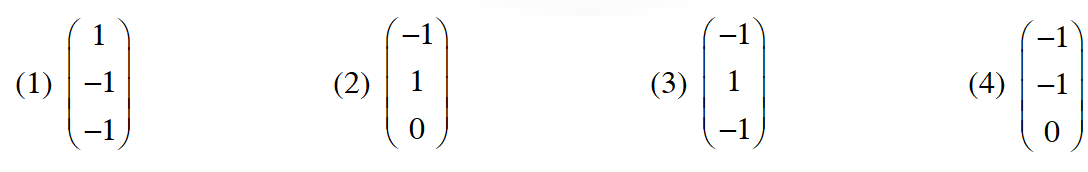[AIEEE-2012]
Ans. (1)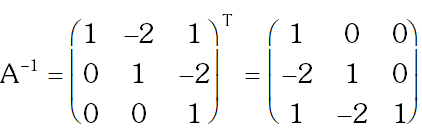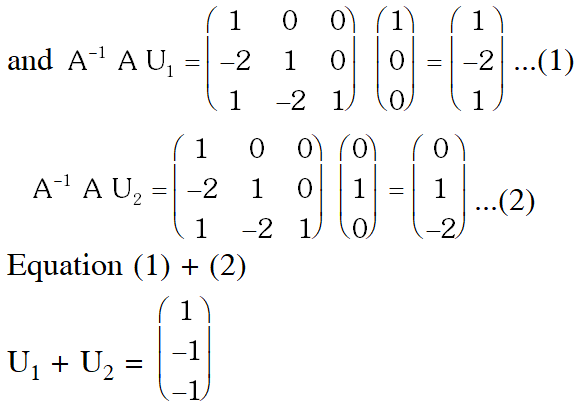Q. If $P=\left[\begin{array}{lll}{1} & {\alpha} & {3} \\ {1} & {3} & {3} \\ {2} & {4} & {4}\end{array}\right]$ is the adjoint of a $3 \times 3$ matrix $A$ and $|A|=4,$ then $\alpha$ is equal to (1) 4                  (2) 11                      (3) 5                     (4) 0 [JEE(Main) - 2013]
Ans. (2) P = adj (A) taking determinant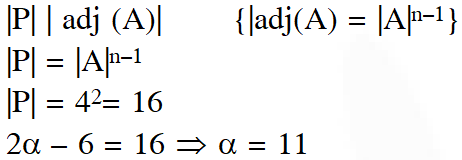Q. If $\mathrm{A}$ is an $3 \times 3 \times 3$ non-singular matrix such that $\mathrm{AA}^{\prime}=\mathrm{A}^{\prime} \mathrm{A}$ and $\mathrm{B}=\mathrm{A}^{-1} \mathrm{A}^{\prime},$ the BB' equals : (1) I + B (2) I (3) $\mathrm{B}^{-1}$ (4) $\left(B^{-1}\right)^{\prime}$ [JEE(Main) - 2014]
Ans. (2)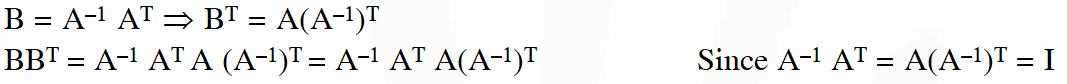Q. If $A=\left[\begin{array}{ccc}{1} & {2} & {2} \\ {2} & {1} & {-2} \\ {a} & {2} & {b}\end{array}\right]$ is a matrix satisfying the equation $A A^{T}=9$, where I is $3 \times 3$ identity matrix, then the ordered pair $(\mathrm{a}, \mathrm{b})$ is equal to : (1) (2, 1)               (2) (–2, –1)                (3) (2, –1)                (4) (–2, 1) [JEE(Main)-2015]
Ans. (2)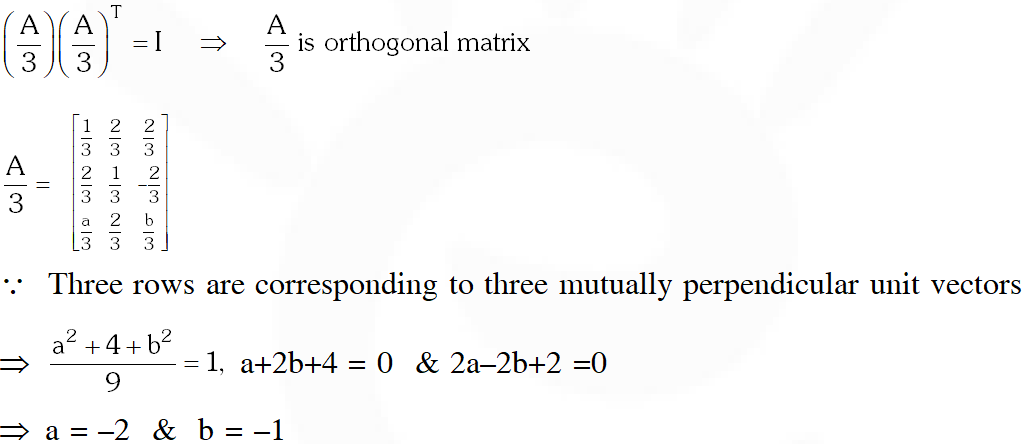Q. If $\mathrm{A}=\left[\begin{array}{cc}{5 \mathrm{a}} & {-\mathrm{b}} \\ {3} & {2}\end{array}\right]$ and $\mathrm{A}$ adj $\mathrm{A}=\mathrm{A} \mathrm{A}^{\mathrm{T}},$ then $5 \mathrm{a}+\mathrm{b}$ is equal to : (1) 13                (2) –1                (3) 5                       (4) 4 [JEE(Main)-2016]
Ans. (3)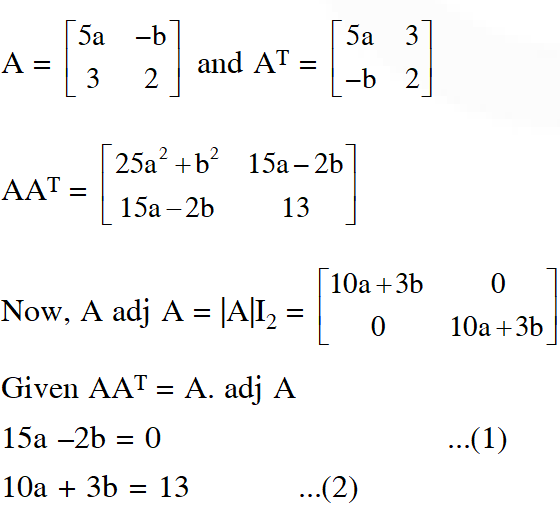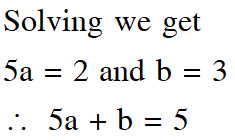Q. If $A=\left[\begin{array}{cc}{2} & {-3} \\ {-4} & {1}\end{array}\right],$ then adj $\left(3 A^{2}+12 A\right)$ is equal to :-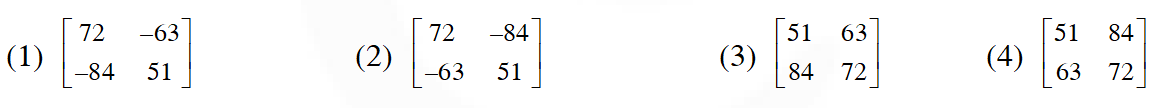[JEE(Main)-2017]
Ans. (3)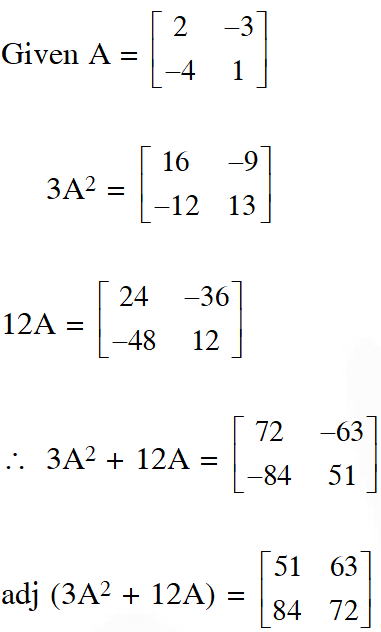Q. Let $\mathrm{A}=\left[\begin{array}{lll}{1} & {0} & {0} \\ {1} & {1} & {0} \\ {1} & {1} & {1}\end{array}\right]$ and $\mathrm{B}=\mathrm{A}^{20} .$ Then the sum of the elements of the first column of $\mathrm{B}$ is : (1) 211               (2) 251               (3) 231                 (4) 210 [JEE(Main)-2018]
Ans. (3)
Q. Let A be a matrix such that A. $\left[\begin{array}{ll}{1} & {2} \\ {0} & {3}\end{array}\right]$ is a scalar matrix and $|3 \mathrm{A}|=108 .$ Then $\mathrm{A}^{2}$ equals :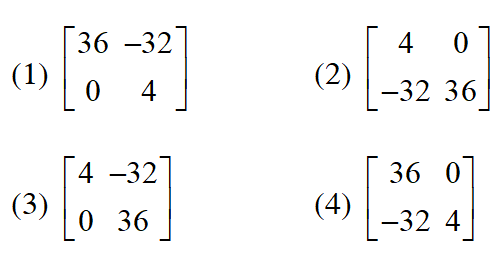[JEE(Main)-2018]
Ans. (1)
Q. Suppose $\mathrm{A}$ is any $3 \times 3$ non-singular matrix and $(\mathrm{A}-3 \mathrm{I})(\mathrm{A}-5 \mathrm{I})=0,$ where $\mathrm{I}=\mathrm{I}_{3}$ and $\mathrm{O}$ $=\mathrm{O}_{3} .$ If $\alpha \mathrm{A}+\beta \mathrm{A}^{-1}=4 \mathrm{I},$ then $\alpha+\beta$ is equal to : (1) 13                 (2) 7                  (3) 12                    (4) 8 [JEE(Main)-2018]
Ans. (4)

Bharath
Aug. 3, 2022, 5:42 a.m.
Don't have sense
Rohan
April 16, 2022, 12:53 a.m.
TATTI E SARAL, E SARAL KI TEAM KE MUH PAR TATTi
E saral Lund
April 16, 2022, 12:52 a.m.
Abbey bsdk JEE ADV ke question ki jagah mains ke de raha hai lodu E SARAL
yumeterou
Sept. 10, 2021, 7:24 p.m.
hiii
esaral jhatu
Sept. 10, 2021, 7:23 p.m.
Terii maa ki chut E-saral, bhosdi ke ye jee main ke question hain na ki advanced, lund e-saral gandu
mr, non iitian
Sept. 8, 2021, 10:53 a.m.
ye sare milke humko pagal bana rahe hain madhachod ke bachhe..............
DC
Sept. 7, 2021, 8:29 p.m.
Gajab chutiya banaya hai.
Rahul
May 14, 2021, 10:27 a.m.
Nikhil Singh
March 16, 2021, 11:54 a.m.
Nice questions
Yui
Feb. 24, 2021, 3:25 a.m.
Fuck
Ninganagoud ayyappagol
Oct. 15, 2020, 10:32 a.m.
I need jee advance questions but hear is jee main quetuons
Munna
Sept. 21, 2020, 7:47 a.m.
Are bevkuf Insan JEE advanced ke question paper chahie Jee main kah de rakha hai yahan par.
Dj
Sept. 19, 2020, 7:49 p.m.
Jee mains ke question de rekhe h aur jee advance ka tag lga rakh h. WoooooooW!
CHANDRA SAILESH
Sept. 15, 2020, 7:34 p.m.
H Urmila
Sept. 15, 2020, 7:32 p.m.
Janu
Sept. 12, 2020, 8:07 p.m.
Sanjay
Sept. 7, 2020, 7:07 p.m.
Asha
Aug. 5, 2020, 12:05 p.m.
You have given most of the questions of jee mains .but title is jee advanced previous years questions.